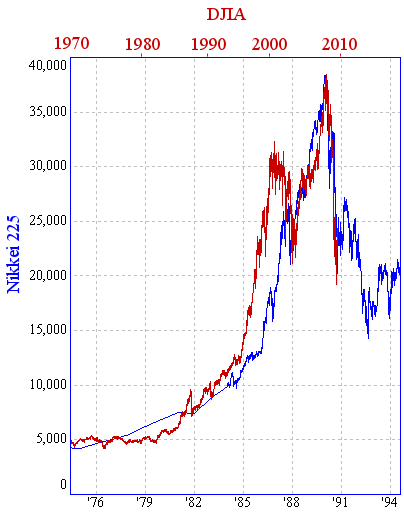Search IntMath
Close

450+ Math Lessons written by Math Professors and Teachers

5 Million+ Students Helped Each Year

1200+ Articles Written by Math Educators and Enthusiasts

Simplifying and Teaching Math for Over 23 Years

# Will the DJIA follow the Nikkei down?

By Murray Bourne, 16 May 2009

Update: See the most recent comparison: DJIA-Nikkei comparison Sep 2010.

I've just finished updating the Dow Jones Model article over on Interactive Mathematics.

It shows how the exponential growth expectations on the Dow Jones Industrial Average may not be panning out as everyone hoped. Instead, the Dow may follow Japan's Nikkei average — down.

One of the many graphs I have in the article is this one, showing that the run-up in values for the US Dow (in red) was quite similar to that of the Nikkei (in blue) 20 years earlier.Will the Dow follow the Nikkei's pattern? Find out more: Dow Jones Model.

Be the first to comment below.

### Comment Preview

HTML: You can use simple tags like <b>, <a href="...">, etc.

To enter math, you can can either:

1. Use simple calculator-like input in the following format (surround your math in backticks, or qq on tablet or phone):
a^2 = sqrt(b^2 + c^2)
(See more on ASCIIMath syntax); or
2. Use simple LaTeX in the following format. Surround your math with $$ and $$.
$$\int g dx = \sqrt{\frac{a}{b}}$$
(This is standard simple LaTeX.)

NOTE: You can mix both types of math entry in your comment.